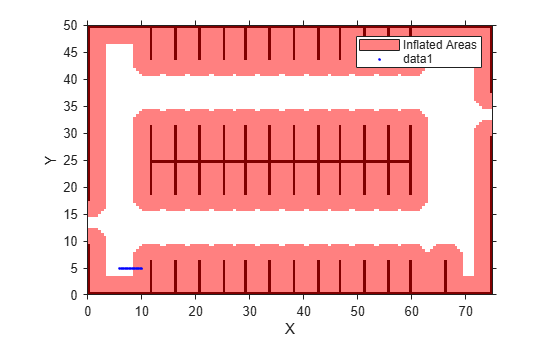# checkOccupied

Check vehicle costmap for occupied poses or points

## Syntax

``occ = checkOccupied(costmap,vehiclePoses)``
``occ = checkOccupied(costmap,xyPoints)``
``occMat = checkOccupied(costmap)``

## Description

The `checkOccupied` function checks whether vehicle poses or points are occupied by obstacles on the vehicle costmap. Path planning algorithms use `checkOccupied` to check whether candidate vehicle poses along a path are navigable.

To simplify the collision check for a vehicle pose, `vehicleCostmap` inflates obstacles according to the vehicle's `InflationRadius`, as specified by the `CollisionChecker` property of the costmap. The collision checker calculates the inflation radius by enclosing the vehicle in a set of overlapping circles of radius R, where the centers of these circles lie along the longitudinal axis of the vehicle. The inflation radius is the minimum R needed to fully enclose the vehicle in these circles. A vehicle pose is collision-free when none of the centers of these circles lie on an inflated grid cell. For more details, see the algorithm on the `vehicleCostmap` reference page.

example

````occ = checkOccupied(costmap,vehiclePoses)` checks whether the vehicle poses are occupied.```
````occ = checkOccupied(costmap,xyPoints)` checks whether (x, y) points in `xyPoints` are occupied.```
````occMat = checkOccupied(costmap)` returns a logical matrix that indicates whether each cell of the costmap is occupied.```

## Examples

collapse all

Load a costmap from a parking lot.

```data = load('parkingLotCostmap.mat'); parkMap = data.parkingLotCostmap; plot(parkMap)```

Create vehicle poses following a straight-line path. `x` and `y` are the (x,y) coordinates of the rear axle of the vehicle. `theta` is the angle of the rear axle with respect to the x-axis. Note that the dimensions of the vehicle are stored in the `vehicleDimensions` property of the costmap, and that there is an offset between the rear axle of the vehicle and its center.

```x = 6:0.25:10; y = repmat(5,size(x)); theta = zeros(size(x)); vehiclePoses = [x',y',theta']; hold on plot(x,y,'b.')```Check if the poses are occupied.

`occ = checkOccupied(parkMap,vehiclePoses)`
```occ = 17x1 logical array 0 0 0 0 0 1 1 1 1 1 ⋮ ```

The vehicle poses are occupied beginning with the sixth pose. In other words, the center of the vehicle in the sixth pose lies within the inflation radius of an occupied grid cell.

## Input Arguments

collapse all

Costmap, specified as a `vehicleCostmap` object.

Vehicle poses, specified as an m-by-3 matrix of [x, y, Θ] vectors. m is the number of poses.

x and y specify the location of the vehicle in world units, such as meters. This location is the center of the rear axle of the vehicle.

Θ specifies the orientation angle of the vehicle in degrees with respect to the x-axis. Θ is positive in the clockwise direction.

Example: `[3.4 2.6 0]` specifies a vehicle with the center of the rear axle at (3.4, 2.6) and an orientation angle of 0 degrees.

Points, specified as an M-by-2 real-valued matrix that represents the (x, y) coordinates of M points.

Example: `[3.4 2.6]` specifies a single point at (3.4, 2.6)

Example: `[3 2;3 3;4 7]` specifies three points: (3, 2), (3, 3), and (4, 7)

## Output Arguments

collapse all

Vehicle pose or point is occupied, returned as an M-by-1 logical vector. An element of `occ` is `1` (`true`) when the corresponding vehicle pose in `vehiclePoses` or planar point in `xyPoints` is occupied.

Costmap cell is occupied, returned as a logical matrix of the same size as the costmap grid. This size is specified by the `MapSize` property of the `costmap`. An element of `occMat` is `1` (`true`) when the corresponding cell in `costmap` is occupied.

## Extended Capabilities

### Functions

Introduced in R2018a